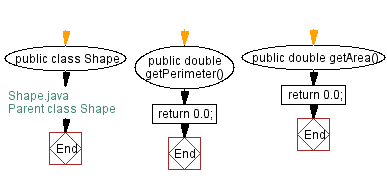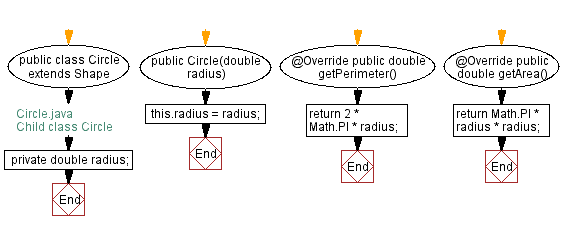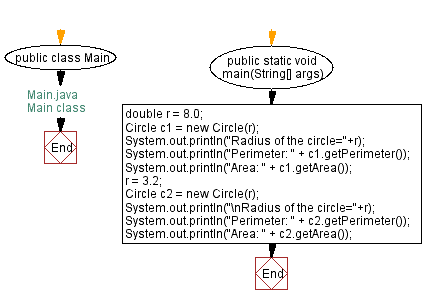﻿ Java Inheritance - Shape class

# Java Inheritance Programming - Create a class called Shape with methods called getPerimeter() and getArea()

## Java Inheritance: Exercise-8 with Solution

Write a Java program to create a class called Shape with methods called getPerimeter() and getArea(). Create a subclass called Circle that overrides the getPerimeter() and getArea() methods to calculate the area and perimeter of a circle.

Sample Solution:

Java Code:

``````// Shape.java
// Parent class Shape

public class Shape {
public double getPerimeter() {
return 0.0;
}

public double getArea() {
return 0.0;
}
}
```
```
``````// Circle.java
// Child class Circle
public class Circle extends Shape {

}

@Override
public double getPerimeter() {
return 2 * Math.PI * radius;
}

@Override
public double getArea() {
}
}
``````
``````// Main.java
// Main class
public class Main {
public static void main(String[] args) {
double r = 8.0;
Circle c1 = new Circle(r);
System.out.println("Perimeter: " + c1.getPerimeter());
System.out.println("Area: " + c1.getArea());
r = 3.2;
Circle c2 = new Circle(r);
System.out.println("Perimeter: " + c2.getPerimeter());
System.out.println("Area: " + c2.getArea());
}
}
``````

Sample Output:

```Radius of the circle=8.0
Perimeter: 50.26548245743669
Area: 201.06192982974676

Perimeter: 20.106192982974676
Area: 32.169908772759484
```

Explanation:

In the above exercise, the Shape class is a base class that provides generic methods for calculating the perimeter and area of a shape. The Circle class is a subclass that extends Shape and overrides the getPerimeter() and getArea() methods to implement circle formulas.

The Circle class has a private radius field that takes a radius argument to initialize the field. The getPerimeter() method uses the formula 2πr to calculate the circumference of the circle. The getArea() method uses the formula πr^2 to calculate the circle area.

In the Main class -

The given code creates two Circle objects, c1 and c2, and calculates their perimeter and area using the getPerimeter() and getArea() methods. The radius of each circle is first set using a double variable r. The output displays the radius, perimeter, and area of each circle.

Flowchart:Java Code Editor:

What is the difficulty level of this exercise?

﻿

## Java: Tips of the Day

How do I remove repeated elements from ArrayList?

If you don't want duplicates in a Collection, you should consider why you're using a Collection that allows duplicates. The easiest way to remove repeated elements is to add the contents to a Set (which will not allow duplicates) and then add the Set back to the ArrayList:

```Set<String> set = new HashSet<>(yourList);
yourList.clear();